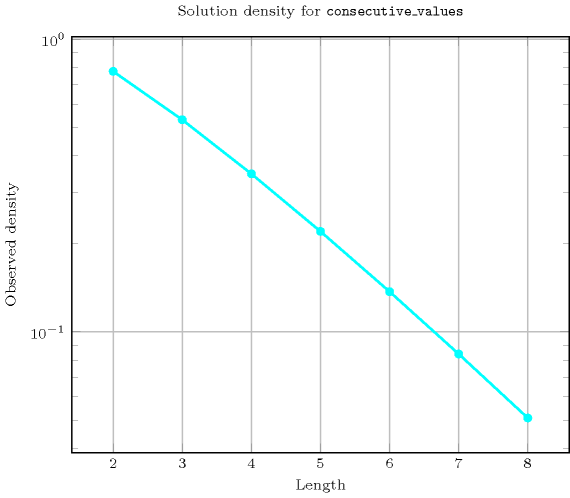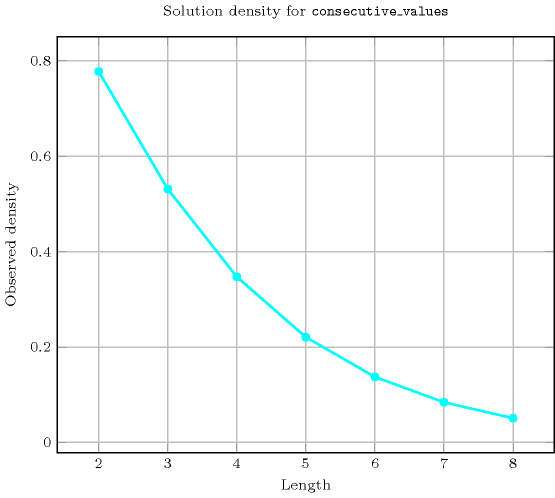## 5.88. consecutive_values

Origin
Constraint

$\mathrm{𝚌𝚘𝚗𝚜𝚎𝚌𝚞𝚝𝚒𝚟𝚎}_\mathrm{𝚟𝚊𝚕𝚞𝚎𝚜}\left(\mathrm{𝚅𝙰𝚁𝙸𝙰𝙱𝙻𝙴𝚂}\right)$

Argument
 $\mathrm{𝚅𝙰𝚁𝙸𝙰𝙱𝙻𝙴𝚂}$ $\mathrm{𝚌𝚘𝚕𝚕𝚎𝚌𝚝𝚒𝚘𝚗}\left(\mathrm{𝚟𝚊𝚛}-\mathrm{𝚍𝚟𝚊𝚛}\right)$
Restriction
$\mathrm{𝚛𝚎𝚚𝚞𝚒𝚛𝚎𝚍}$$\left(\mathrm{𝚅𝙰𝚁𝙸𝙰𝙱𝙻𝙴𝚂},\mathrm{𝚟𝚊𝚛}\right)$
Purpose

Constraint the difference between the largest and the smallest values of the $\mathrm{𝚅𝙰𝚁𝙸𝙰𝙱𝙻𝙴𝚂}$ collection to be equal to the number of distinct values assigned to the variables of the $\mathrm{𝚅𝙰𝚁𝙸𝙰𝙱𝙻𝙴𝚂}$ collection minus one (i.e., there is no holes at all within the used values).

Example
$\left(〈5,4,3,5〉\right)$

The $\mathrm{𝚌𝚘𝚗𝚜𝚎𝚌𝚞𝚝𝚒𝚟𝚎}_\mathrm{𝚟𝚊𝚕𝚞𝚎𝚜}$ constraint holds since all values between value 3 and value 5 are actually used.

Typical
 $|\mathrm{𝚅𝙰𝚁𝙸𝙰𝙱𝙻𝙴𝚂}|>1$ $\mathrm{𝚛𝚊𝚗𝚐𝚎}$$\left(\mathrm{𝚅𝙰𝚁𝙸𝙰𝙱𝙻𝙴𝚂}.\mathrm{𝚟𝚊𝚛}\right)>1$
Symmetries
• Items of $\mathrm{𝚅𝙰𝚁𝙸𝙰𝙱𝙻𝙴𝚂}$ are permutable.

• One and the same constant can be added to the $\mathrm{𝚟𝚊𝚛}$ attribute of all items of $\mathrm{𝚅𝙰𝚁𝙸𝙰𝙱𝙻𝙴𝚂}$.

Counting
 Length ($n$) 2 3 4 5 6 7 8 Solutions 7 34 217 1716 16159 176366 2187637

Number of solutions for $\mathrm{𝚌𝚘𝚗𝚜𝚎𝚌𝚞𝚝𝚒𝚟𝚎}_\mathrm{𝚟𝚊𝚕𝚞𝚎𝚜}$: domains $0..n$$\mathrm{𝚌𝚘𝚗𝚜𝚎𝚌𝚞𝚝𝚒𝚟𝚎}_\mathrm{𝚟𝚊𝚕𝚞𝚎𝚜}\left(\mathrm{𝚅𝙰𝚁𝙸𝙰𝙱𝙻𝙴𝚂}\right)$
with  $|\mathrm{𝚅𝙰𝚁𝙸𝙰𝙱𝙻𝙴𝚂}|>$$\mathrm{𝚛𝚊𝚗𝚐𝚎}$$\left(\mathrm{𝚅𝙰𝚁𝙸𝙰𝙱𝙻𝙴𝚂}.\mathrm{𝚟𝚊𝚛}\right)$
implies $\mathrm{𝚜𝚘𝚖𝚎}_\mathrm{𝚎𝚚𝚞𝚊𝚕}$$\left(\mathrm{𝚅𝙰𝚁𝙸𝙰𝙱𝙻𝙴𝚂}\right)$.